# “CutMix: Regularization Strategy to Train Strong Classifiers with Localizable Features” Summarized

Categories:

Updated:

https://arxiv.org/abs/1905.04899 (2019-08-07)

## 1. Cutout + Mixup = CutMix

### Cutout

To prevent a CNN from focusing too much on a small set of intermediate activations or on a small region on input images, random feature removal regularizations have been proposed.

• Dropout: randomly drop hidden activations
• Regional dropout: erasing random regions on the input

Well known strategy for regional dropout is ‘Cutout’, where you randomly replace random region from images with zeros or noise, before feeding them in to the model.

Cutout lets models attend not only to the most discriminative parts of objects, but rather to the entire object region.

However, applying cutout greatly reduces the proportion of informative pixels on training images.

### Mixup

Mixup is another augmentation strategy to make a generalizable model. It ‘mixes’ two random images $x_a$ and $x_b$ from a batch ($\lambda x_a + (1-\lambda)x_b$) and set it’s label to $\lambda y_a + (1-\lambda)y_b$.

However Mixup samples suffer from the fact that they are locally ambiguous and unnatural, and therefore confuses the model, especially for localization.

### CutMix

The authors introduce an augment strategy called ‘CutMix’. Instead of simply removing pixels, it replaces the removed regions with a patch from another image. The ground truth labels are also mixed proportionally to the number of pixels of combined images.

With CutMix, the model do not have any uninformative pixel(zero or noise) during training, while retaining the advantages of regional dropout to attend to non-discriminative parts of objects. The added patches further enhance localization ability, by requiring the model to identify the object from a partial view.

Although Mixup and Cutout enhance ImageNet classiﬁcation accuracy, they decrease the ImageNet localization or object detection performances. On the other hand, CutMix consistently achieves signiﬁcant enhancements across three tasks.

Below is an overview of the results of three techniques on ImageNet classification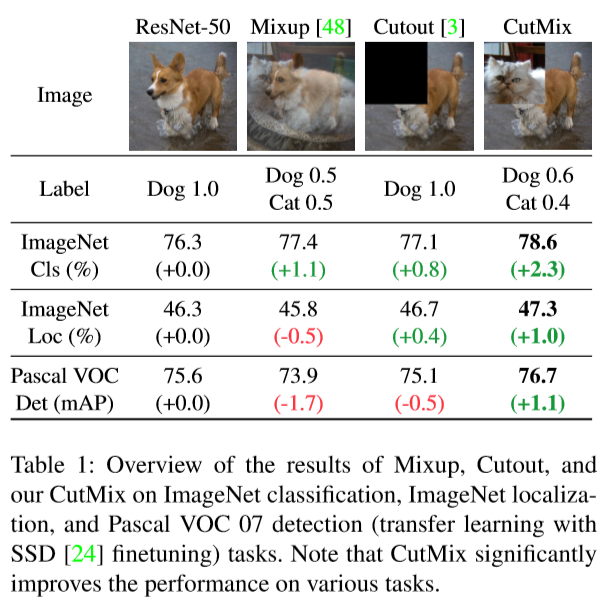## 2. CutMix Algorithm

First, we randomly select two images, $x_A$ and $x_B$ from a mini-batch. Then we generate bounding box coordinates $\bf{B}$ $= (r_x, r_y, r_w, r_h)$ indicating the cropping regions on $x_A$ and $x_B$. The regions $\bf{B}$ in $x_A$ is removed and filled in with the patch cropped from $\bf{B}$ of $x_B$. $\bf{B}$ is generated with the following formula.

In their experiments, the authors set $\alpha$ to 1, so that combination ratio $\lambda$ is sampled from the uniform distribution (0,1).

This ensures two things.

• Cropped area ratio $\frac{r_wr_h}{WH}=1-\lambda$.
• Cropped width/height ratio is the same as the original width/height ratio.

Then, we will generate a new training sample $(\tilde{x}, \tilde{y})$ from original sample $(x,y)$ using binary mask $\bf{M}$ generated from bounding box $\bf{B}$.

Below is a pseudo-code for CutMix.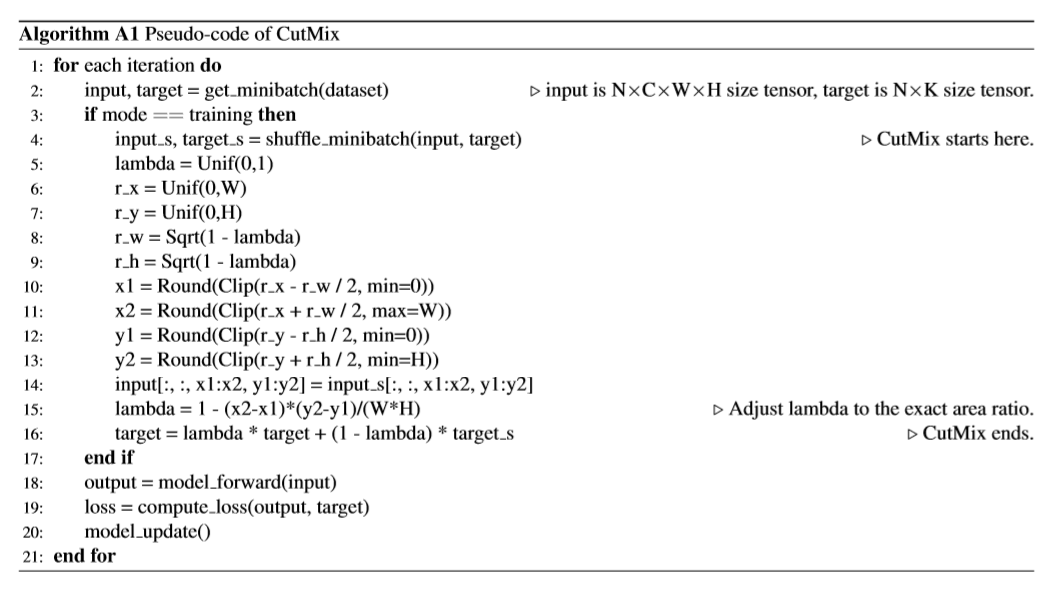## 3. Learning Spatially Distributed Representation

### Cam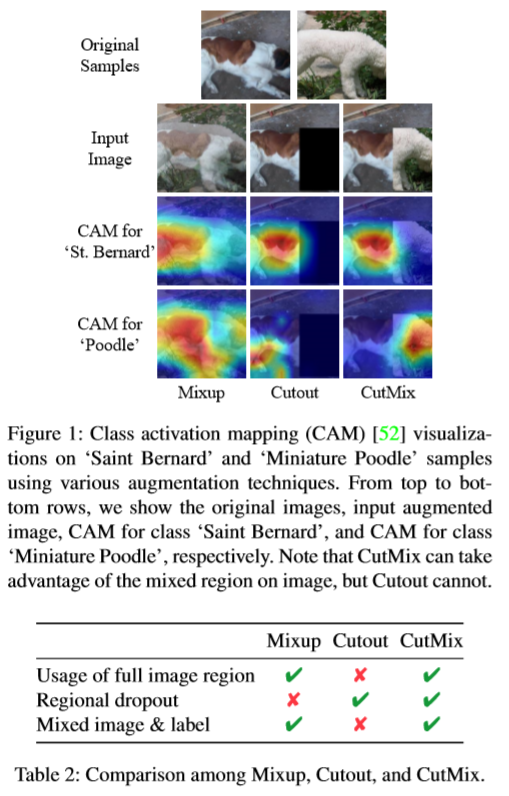Cutout successfully lets a model focus on less discriminative parts of the object, such as the belly of Saint Bernard, while being inefficient due to unused pixels. Mixup, on the other hand, makes full use of pixels, but introduces unnatural artifacts. The CAM for Mixup, as a result, shows that the model is confused when choosing cues for recognition, which leads to its suboptimal performance in classification and localization. On the other hand, CutMix efficiently improves upon cutout by being able to localize the two object classes accurately.

### WSOL

Weakly supervised object localization (WSOL) task aims to train the classifier to localize target objects by using only the class labels. To localize the target well, it is important to make CNNs extract cues from full object regions and not focus on small discriminant parts of the target. CutMix guides a classifier to attend to broader sets of cues to make decisions, and resulted in superior performance.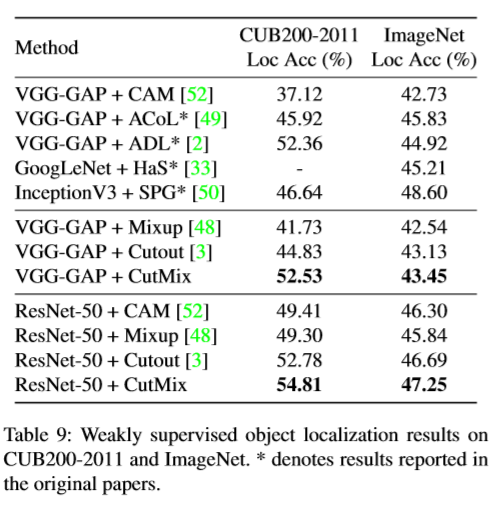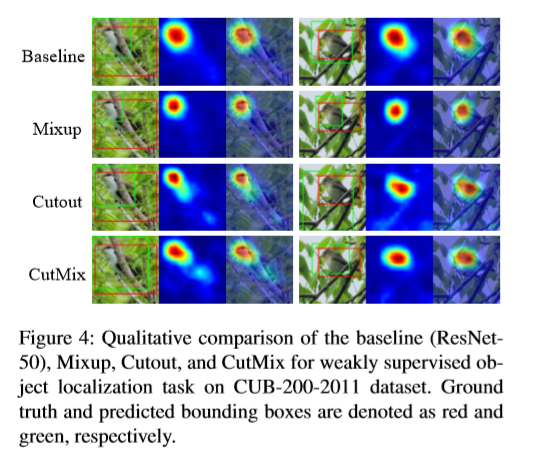## 4. Robustness to Adversarial Attacks

CutMix significantly improves the robustness to adversarial attacks compared to other augmentation methods.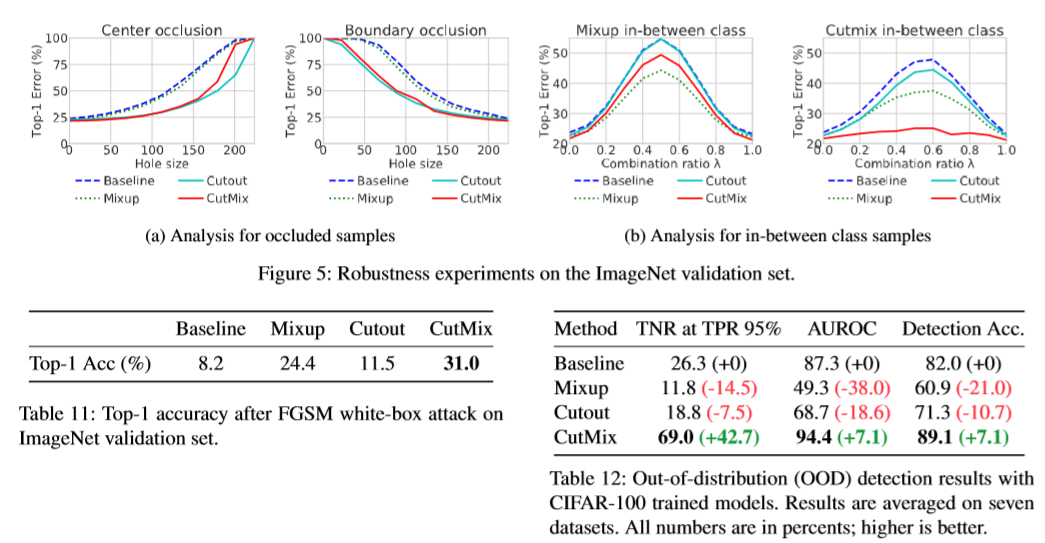## 5. Experiment Results on ImageNet and CIFAR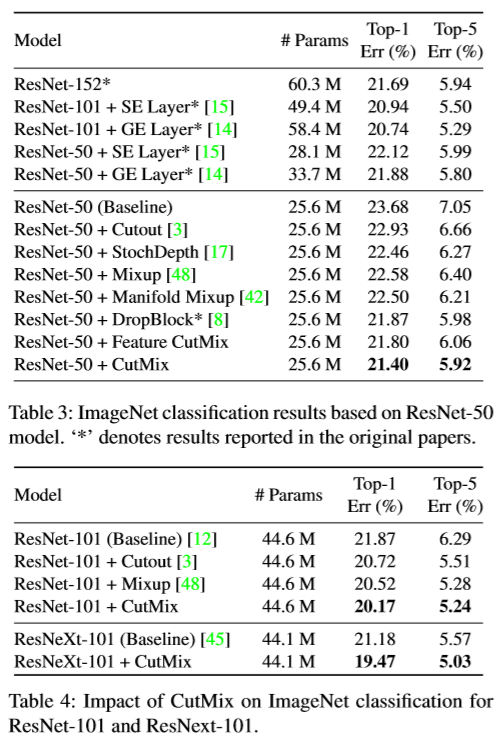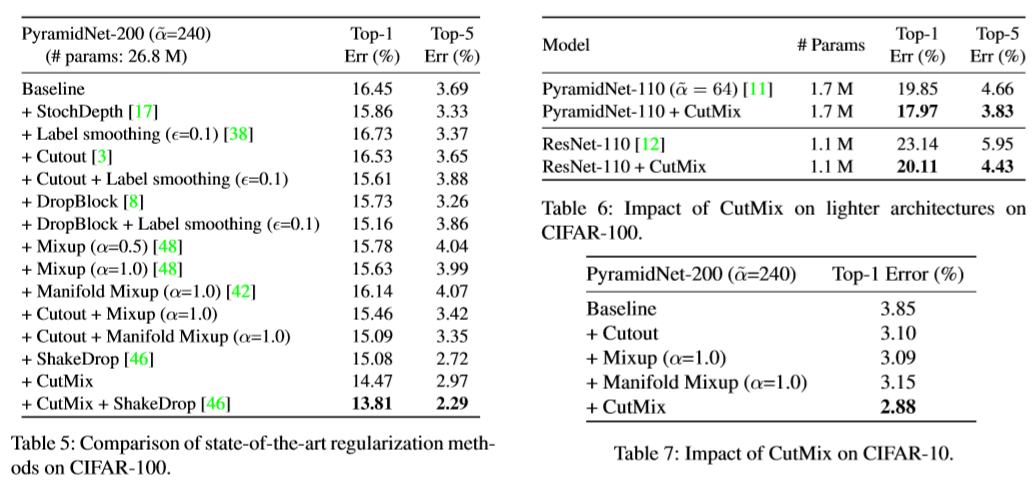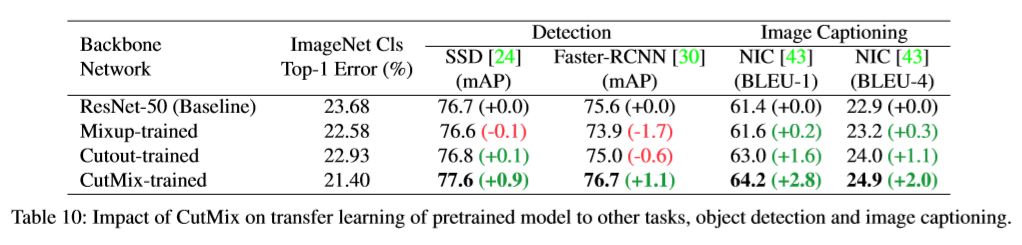## 6. Variants of CutMix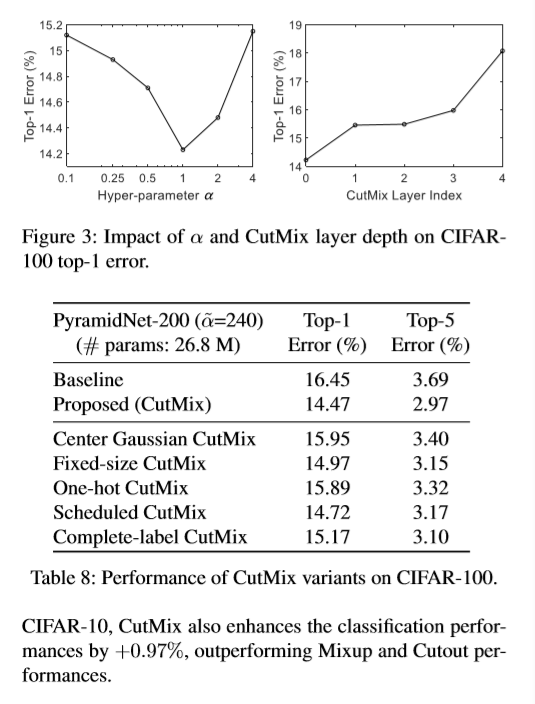• Center Gaussian CutMix: It samples the box coordinates $r_x, r_y$ according to the Gaussian distribution
• Fixed-size CutMix: It fixes the size of cropping region $r_w, r_h$ at 16x16.
• Scheduled CutMix: It linearly increases the probability to apply CutMix as training progresses.
• One-hot CutMix: It decides the mixed target label by committing to the label of greater patch portion.
• Complete-label CutMix: It assigns the mixed target label as $\tilde{y}=0.5y_A+0.5y_B$ regardless of the combination ratio $\lambda$.
• Different $\alpha$ for Beta distribution, and different layer index to apply cutmix.

The results show that above variants lead to performance degradation compared to the original CutMix.

Categories: